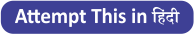Latest Banking jobs   »   Reasoning Ability Quiz For SBI PO,...

# Reasoning Ability Quiz For SBI PO, Clerk Prelims 2021- 13th June

Directions (1-5): In these questions, relationship between different elements is show in the statements. The statements are followed by conclusions. Study the conclusions based on the given statements and select the appropriate answer:
(a) If only conclusion I follows.
(b) If only conclusion II follows.
(c) If either conclusion I or II follows
(d) If neither conclusion I nor II follows.
(e) If both conclusions I and II follow.

Q1. Statements: U>I>X<M≤P<L=E≥R<T
Conclusion I: U>M II: E>M

Q2. Statements: W>R=T>Y<M<N≤X=D>F
Conclusion I: D≥N II: W>Y

Q3. Statements: S≥C>M≤O>L=P>R<T>H
Conclusion I: S>L II: O>T

Q4. Statements: X<M=U≥L>J=K>F<G≤R≥Y
Conclusion I: L≤R II: R>L

Q5. Statements: Z>W>T>D≤M<L≥I>O≥P=Q
Conclusion I: M>Q II: D<L

Directions (6-8): In each of the question, relationships between some elements are shown in the statements(s). These statements are followed by conclusions numbered I and II. Read the statements and give the answer.
(a) If only conclusion I follows.
(b) If only conclusion II follows.
(c) If either conclusion I or II follows.
(d) If neither conclusion I nor II follows.
(e) If both conclusions I and II follow.

Q6. Statements: C < M >N = T, C ≤ V, M > R
Conclusions: I. N > R II. V > T

Q7. Statements: C ≥ D< T = F ≥ G, C < W
Conclusions: I. T =G II. G< T

Q8. Statements: R < T < S < P > Q, R> X
Conclusions: I. S < Q II. X < S

Directions (9-11): In these questions, relationship between different elements is show in the statements. The statements are followed by conclusions. Study the conclusions based on the given statements and select the appropriate answer:
(a) If only conclusion I follows.
(b) If only conclusion II follows.
(c) If either conclusion I or II follows
(d) If neither conclusion I nor II follows.
(e) If both conclusions I and II follow.

Q9. Statements: H>S>T<V> D, M<T>U
Conclusion I: V>M II: H>U

Q10. Statements: R>E≥V=G≥S, T<V≤W
Conclusion I: S=W II: W>S

Q11. Statements: D>G<Y>Q, P>Y, G>T
Conclusion I: Q>T II: T<P

Q12. In which of the following expression will the expression ‘G < A’ holds true?
(a) G≤E=H>A≤D
(b) H>E=D<A≥B=G
(c) A<B=D≥E=G
(d) E<G≥D>B≥A=H
(e) G≤D<E≤A<B

Q13. if the given expression ‘A<B≥C>D=E’ is definitely true, then which of the following expressions will be true?
(a) A<D
(b) E≥B
(c) C≤E
(d) E<A
(e) None is true

Q14. If the expressions, ‘W>U≤X<T,’V≥X’ and ‘X≥Z’ are true then which of the following combinations will be definitely true?
(a) U ≥ Z
(b) W < Z
(c) V = Z
(d) U ≤ V
(e) None of these

Q15. Which of the following symbols should replace the sign (#) and (&) respectively in the given expression in order to make the expression E < F and C ≥ D definitely true?
B < E ≤ G = H < D # F ≤ A & C
(a) ≥, =
(b) ≥, ≤
(c) >, ≤
(d) =, ≤
(e) ≥, <

Practice More Questions of Reasoning for Competitive Exams:

###### SBI Clerk Study Plan 2021

SolutionsSolution (1-5):
S1. Ans(b)
Sol. I: U>M(False) II: E>M(True)

S2. Ans(e)
Sol. I: D≥N(True) II: W>Y(True)

S3. Ans(d)
Sol. I: S>L(False) II: O>T(False)

S4. Ans(d)
Sol. I: L≤R(False) II: R>L(False)

S5. Ans(b)
Sol. I: M>Q(False) II: D<L(True)

Solutions (6-8):
S6. Ans.(d)
Sol. I. N > R (False) II. V > T (False)

S7. Ans.(c)
Sol. I. T =G (False) II. G < T (False)

S8. Ans.(b)
Sol. I. S < Q (False) II. X < S (True)

Solution (9-11):
S9. Ans(e)
Sol. I: V>M(True) II: H>U(True)

S10. Ans(c)
Sol. I: S=W(False) II: W>S(False)

S11. Ans(b)
Sol. I: Q>T(False) II: T<P(True)

S12. Ans.(e)

S13. Ans.(e)

S14. Ans.(d)

S15. Ans.(d)